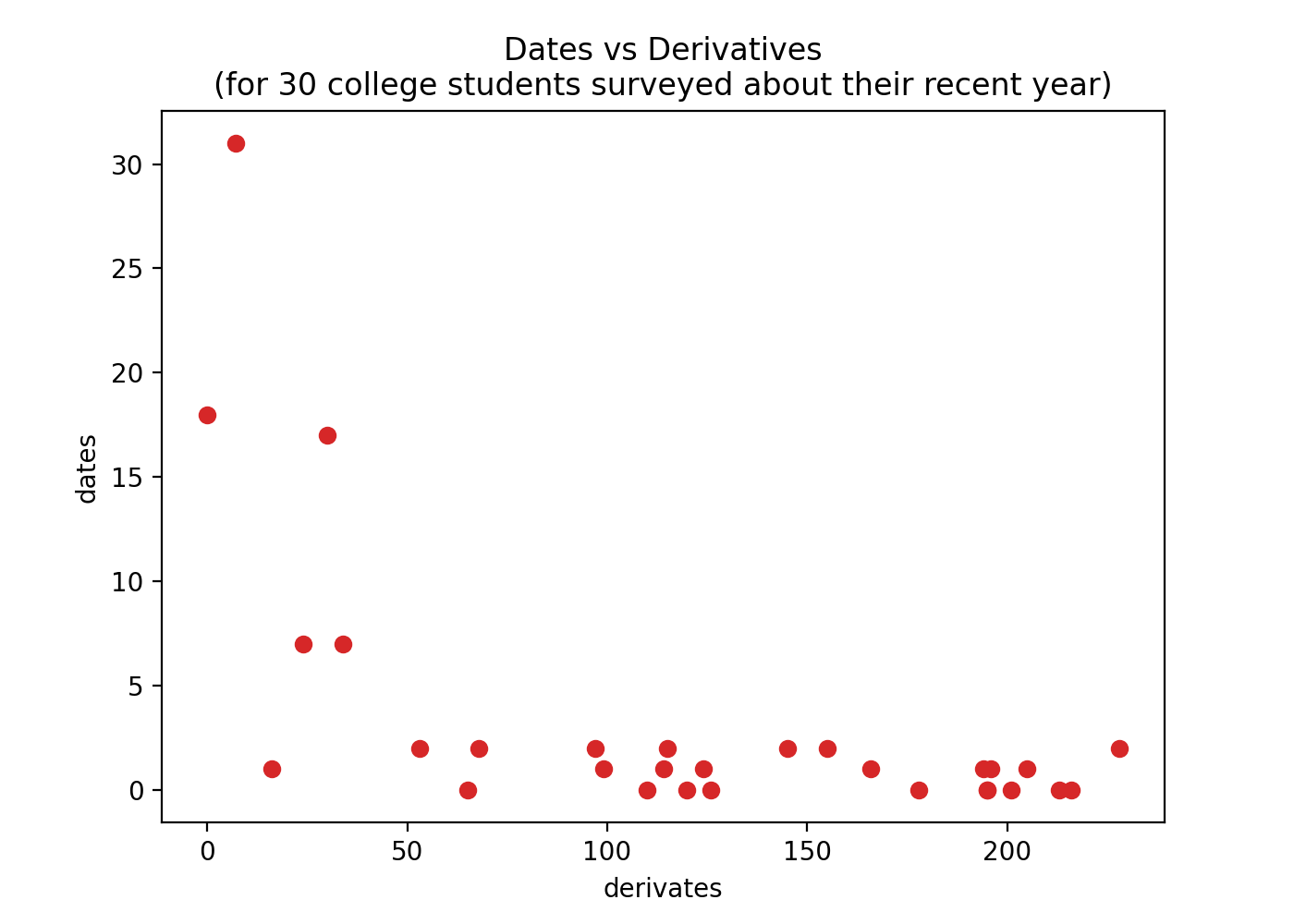# Dates vs Derivatives Problem¶

Thirty college students were surveyed on

1. the number of derivatives they've calculated in the past year and
2. the number of dates they've been on in the past year
import random

random.seed(1)
derivatives = [random.randrange(0, 250) for i in range(30)]
dates = [random.randrange(0, max(50 - d, 3)) for d in derivatives]

print(derivatives)
# [34, 145, 216, ..., 178, 114, 68]

print(dates)
# [7, 2, 0, ..., 0, 1, 2]


Build a scatter plot of this data with

• derivatives on the x axis and dates on y axis (with labeled axes)
• points using the color 'tab:red'
• the title:

Dates vs Derivatives
(for 30 college students surveyed about their recent year)

Show the plot